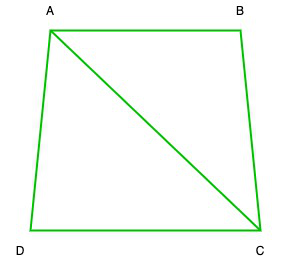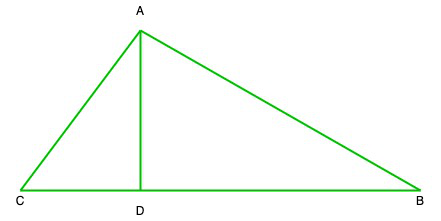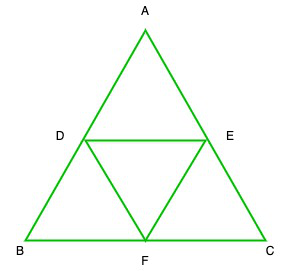Related Articles

# Class 10 RD Sharma Solutions – Chapter 14 Coordinate Geometry – Exercise 14.5 | Set 1

• Last Updated : 16 May, 2021

### (i) (6, 3), (-3, 5) and (4, -2)

Solution:

Let us assume ABC is a triangle whose vertices are A(6, 3), B(-3, 5) and C(4, -2)

So,

Area of ∆ABC = 1/2[x1(y2 – y3) + (y3 – y1)x2 + x3(y1 – y2)]

= 1/2[6(5 + 2) + (-3)(-2 – 3) + 4(3 – 5)]

= 1/2[6(5 + 2) + (-3)(-2 – 3) + 4(3 – 5)]

= 1/2[42 + 15 – 8]

= 1/2 * 49

= 49/2 sq.unit

### (ii) (at12, 2at1), (at22, 2at2) and (at32, 2at3)

Solution:

Let us assume ABC is a triangle whose vertices are A(at12, 2at1), (at22, 2at2) and (at32, 2at3

Area of ∆ABC = 1/2[x1(y2 – y3) + (y3 – y1)x2 + x3(y1 – y2)]

= 1/2[at12(2at2 – 2at3) + at22(2at3 – 2at1) + at32(2at1 – 2at2)]

= 1/2[at122at2 – at122at3 + at222at3 – at222at1 + at322at1 – at322at2]

= 1/2 × 2a2[t12t2 – t12t3 + t22t3 – t22t1 + t32t1 – t32t2]

= a2[t12(t2 – t3) + t22(t3 – t1) + t32(t1 – t2)]

### (iii) (a, c + a), (a, c) and (-a, c – a)

Let us assume ABC is a triangle whose vertices are A(a, c + a), B(a, c) and C(-a, c – a)

So, Area of ∆ABC = 1/2[x1(y2 – y3) + (y3 – y1)x2 + x3(y1 – y2)]

= 1/2[a(c – c + a)a(c – a – c – a) + (-a)(c + a – c)]

= 1/2(a2 – 2a2 – a2)

= 1/2(-2a2)

= a2sq.units

### (i) (-3, 2), (5, 4), (7, -6) and (-5, -4)

Solution:Let us considered ABCD is a quadrilateral whose vertices are A(-3, 2), B(5, 4), C(7, -6) and D(-5, -4)

So, Area of ABCD = Area of ∆ABC + Area of ∆ADC   ….(1)

So,

So, Area of ∆ABC = 1/2[x1(y2 – y3) + (y3 – y1)x2 + x3(y1 – y2)]

= 1/2 [-3(4 + 6) + (-6 – 2) + 7(2 – 4)]

= 1/2 [-3(10) + 5 * (-8) + 7 * (-2)]

= 1/2 [-30 – 40 – 14]

= 1/2 [-30-40-14]

= 1/2 (-84)

= -42

= 42 sq.units (Area cannot be in negative)

= 1/2 [-3(-6 + 4) + 7(-4 – 2) + (-5)(2 + 6)]

= 1/2 [-3(-2) + 7(-6) + (-5)(8)]

= 1/2 [6 – 42 – 40]

= 1/2 (-76)

= -38

= 38 sq. Units(Area cannot be in negative)

Now put all these values in eq(1), we get

Area of quadrilateral ABCD = 42 + 38 = 80 sq.units

### (ii) (1, 2), (6, 2), (5, 3) and (3, 4)

Solution:Let us considered ABCD is a quadrilateral whose vertices are A(1, 2), B(6, 2), C(5, 3) and D(3, 4)

So, Area of ABCD = Area of ∆ABC + Area of ∆ADC   ….(1)

So, Area of ∆ABC = 1/2[x1(y2 – y3) + (y3 – y1)x2 + x3(y1 – y2)]

= 1/2 [1(2 – 3) + 6(3 – 2) + 5(2 – 2)]

= 1/2[-1 + 6 + 0]

= 1/2 (5)

= 5/2 sq.units

= 1/2 [1(3 – 4) + 5(4 – 2) + 3(2 – 3)]

= 1/2 [1 * (-1) + 5 * 2 + 3 * (-1)]

= 1/2 [-1 + 10 – 3]

= 1/2 * 6

= 3 sq.units

Now put all these values in eq(1), we get

Area of quadrilateral ABCD = 5/2 + 3 = (5 + 6)/2 = 11/2 sq.units

### (iii) (-4, -2), (-3, -5), (3, -2), (2, 3)

Solution:Let us considered ABCD is a quadrilateral whose vertices are A(-4, -2), B(-3, -5), C(3, -2), D(2, 3)

So, Area of ABCD = Area of ∆ABC + Area of ∆ADC   ….(1)

So, Area of ∆ABC = 1/2[x1(y2 – y3) + (y3 – y1)x2 + x3(y1 – y2)]

= 1/2 [-4(-5 + 2) – 3(-2 + 2) + 3(-2 + 5)]

= 1/2 [-4 * (-3) – 3 * 0 + 3 * 3]

= 1/2 [12 + 0 + 9]

= 1/2 * 21

= 21/2 sq.units

= 1/2 [-4(-2 – 3) + 3(3 + 2) + 2(-2 + 2)]

= 1/2[-4 * (-5) + 3 * 5 + 2 * 0]

= 1/2 [20 + 15 + 0]

= 1/2 * 35

= 35/2 sq.units

Now put all these values in eq(1), we get

Area of quadrilateral ABCD = 21/2 + 35/2 + 56/2 = 28 sq.units

### Question 3. The four vertices of a quadrilateral are (1, 2), (-5, 6), (7, -4) and (k, -2) taken in order. If the area of the quadrilateral is zero, find the value of k?

Solution:Let us considered ABCD is a quadrilateral whose vertices are A(1, 2), B(-5, 6), C(7, -4) and D(k, -2)

So, Area of ABCD = Area of ∆ABC + Area of ∆ADC   ….(1)

Now area of ∆ABC

= 1/2[x1(y2 – y3) + (y3 – y1)x2 + x3(y1 – y2)]

= 1/2 [1(6 + 4) + (-5)(-4 – 2) + 7(2 – 6)]

= 1/2 [1 * 10 + (-5)(-6) + 7(-4)]

= 1/2 [10 + 30 – 28]

= 1/2 × 12

= 6sq.units

= 1/2 [1(-4 + 2) + 7(-2 – 2) + k(2 + 4)]

= 1/2 [1(-2) + 7(-4) + k(-4)]

= 1/2 [-2 – 28 + 6k]

= 1/2 (-30 + 6k)

= -15+3k

= 3k – 15 sq.units

Now put all these values in eq(1), we get

Area of quadrilateral ABCD = 6 + 3k – 15

But it is given that the area of quadrilateral ABCD = 0

So,

0 = 6 + 3k – 15

k = 9/3 = 3

Hence, the value of k is 3.

### Question 4. The vertices of ∆ABC are (-2, 1), (5, 4), and (2, -3) respectively. Find the area of the triangle and the length of the altitude through A.

Solution:Given that, ABC is a triangle whose vertices are A(-2, 1), B(5, 4), and C(2, -3)

So, area of ∆ABC = 1/2[x1(y2 – y3) + (y3 – y1)x2 + x3(y1 – y2)]

= 1/2 [-2(4 + 3) + 5(-3 – 1) + 2(1 – 4)]

= 1/2 [-2 * 7 + 5 * (-4) + 2(-3)]

= 1/2[-14 – 20 – 6]

= 1/2 * (-40)

= -20

= 20 sq. units

Now we find the length of BC == √58 units

So, the area of ∆ABC = 1/2 * base * height

⇒ 20 = 1/2 * 58 * h

⇒ h = (20 * 2)/√58 = 40/√58 units

### (i) (2, 5), (4, 6) and (8, 8)

Solution:

As we know that 3 points are collinear is the area of the triangle formed by them is zero

So, let us assume ABC is a triangle whose vertices A(2, 5), B(4, 6) and C(8, 8)

So, area of ∆ABC = 1/2[x1(y2 – y3) + (y3 – y1)x2 + x3(y1 – y2)]

= 1/2[2(6 – 8 + 4(8 – 5) + 8(5 – 6)]

= 1/2 [2 * (-2) + 4 * 3 + 8 * (-1)]

= 1/2 [-4 + 12 – 8]

= 1/2 * 0 = 0

Area of ∆ABC = 0

Hence, points A, B and C are collinear

Hence proved

### (b) (1, -1), (2, 1) and (4, 5)

Solution:

As we know that 3 points are collinear is the area of the triangle formed by them is zero

So, let us assume ABC is a triangle whose vertices A(1, -1), B(2, 1) and C(4, 5)

So, area of ∆ABC = 1/2[x1(y2 – y3) + (y3 – y1)x2 + x3(y1 – y2)]

= 1/2 [1(1 – 5) + 2(5 + 1) + 4(-1 – 1)]

= 1/2 [1(-4) + 2 * 6 + 4(-2)]

= 1/2 [-4 + 12 – 8]

= 1/2 * 0 = 0

Area of ∆ABC = 0

Hence, points A, B and C are collinear

Hence proved

### Question 6. Find the area of a quadrilateral ABCD, the coordinates of whose vertices are A (-3, 2), B (5, 4), C (7, -6), and D (-5, -4).

Solution:

Given that, ABCD is a quadrilateral whose vertices A (-3, 2), B (5, 4), C (7, -6), and D (-5, -4).

So, Area of quadrilateral ABCD = area of ∆ABC + area of ∆ACD

= 1/2|(-12 – 30 + 14) – (10 + 28 + 18)| + 1/2|(18 – 28 – 10) – (14 + 30 + 12)|

= 1/2|-28 – 56| + 1/2|-20 – 56|

= 1/2|-84| + 1/2|-76|

= 42 + 38

= 80 sq.units

Hence, the area of quadrilateral is 80 sq.units

### Question 7. In ∆ABC, the coordinates of vertex A are (0, -1) and D (1, 0) and E (0, 1) respectively the mid-points of the sides AB and AC. If F is the mid-point of side C, find the area of ∆DEF.

Solution:Given that ∆ABC, the coordinates of vertex A are (0, -1) and D (1, 0) and E (0, 1) are

the mid points of the sides AB and AC

Let us considered B (p, q), C (r, s) and F (x, y)

So, Mid-point of AB = Coordinates of Dp/2 = 1

p = 2

Therefore, B(p, q) = B(2, 1)

(-1 + q)/2 = 0

-1 + q = 0

q = 1

Mid-point of AC = coordinates of Er/2 = 0

r = 0

(-1 + s)/2 = 1

-1 + s = 2

s = 3

Now, C(r, s) = C(0, 3)

Coordinates of F = Midpoint of BCSo, the coordinates of A(0,-1), B(2, 1) and C(0, 3)

Area of ∆ABC = 1/2[x1(y2 – y3) + (y3 – y1)x2 + x3(y1 – y2)]

= 1/2 [0(1 – 3) + 2(3 + 1) + 0(-1 – 1)]

= 1/2(8) = 4sq.units

And the coordinate of D(1, 0), E(0, 1), and F(1, 2)

Area of ∆DEF = 1/2[1(1 – 2) + 0(2 – 0) + 1(0 – 1)]

= 1/2(-1 – 1) = -1

= 1 sq.unit (Area cannot be in negative)

### Question 8. Find the area of the triangle PQR with Q (3, 2) and the mid-points of the sides through Q being (2, -1) and (1, 2).

Solution:

Given that, ∆PQR, L and N are mid-points of QR and QP, and the coordinates of Q are (3, 2) of L are (2, -1) and of N are (1, 2)

Let us assume that the coordinates of R be (x1, y1) and P(x2,y2)

2 = (3 + x1)/2 ⇒ 3 + x1 = 4 ⇒ x1 = 4 – 3 = 1

and -1 = (2 + y)/2 ⇒ -2 = 2 + y ⇒ y = -2 – 2 = -4

The coordinates of R are (1,-4)

Similarly coordinates of P will be

1 = (3 + x1)/2 ⇒ 3 + x1 = 2 ⇒ x1 = 2 – 3 = -1

and (2 + y)/1 = 2 ⇒ 2 + y = 4 ⇒ y = 4 – 2 = 2

Coordinates of P are (-1, 2)

Now area of ∆PQR

= 1/2 [(-12 + 2 – 2) – (2 + 4 + 6)]

= 1/2[-12 – 12] = -12

= 12 sq.units

### Question 9. If P (-5, -3), Q (-4, -6), R (2, -3) and S (1, 2) are the vertices of a quadrilateral PQRS, find its area.

Solution:

Given that, PQRS quadrilateral whose vertices are P (-5, -3), Q (-4, -6), R (2, -3) and S (1, 2)

Area of PQRS quadrilateral = Area of ∆PQR + Area of ∆PSR   ….(1)

Now area of ∆PQR = 1/2[x1(y2 – y3) + (y3 – y1)x2 + x3(y1 – y2)]

= 1/2[-5(-6 + 3) + (-4)(-3 + 3) + 2(-3 + 6)]

= 1/2[-5 * (-3) + (-4 * 0) + 2 * 3]

= 1/2(15 + 0 + 6)

= 1/2 * 21

= 21/2

Similarly the area ∆PSR,

= 1/2[-5 * (2 + 3) + 1(-3 + 3) + 2(-3 – 2)]

= 1/2[-5 * 5 + 1 * 0 + 2 * (-5)]

= 1/2[-25 + 0 – 10]

= 1/2 * (-35)

= -35/2

Now put all these values in eq(1), we get

Area of quadrilateral PQRS = 21/2 + (-35/2) = 28 sq.unit

### Question 10. If A (-3, 5), B (-2, -7), C (1, -8), and D (6, 3) are the vertices of a quadrilateral ABCD, find its area.

Solution:

Let us considered ABCD is a quadrilateral whose vertices areA (-3, 5), B (-2, -7), C (1, -8), and D (6, 3)

So, Area of ABCD = Area of ∆ABC + Area of ∆ACD   ….(1)

So, Area of ∆ABC = 1/2[x1(y2 – y3) + (y3 – y1)x2 + x3(y1 – y2)]

= 1/2 [-3(-7 + 8) + (-2)(-8 – 5) + 1(5 + 7)]

= 1/2 [-3 * 1 + 26 + 12]

= 1/2[-3 + 26 + 12]

= 35/2 sq.units

Similarly, area of ∆ACD = 1/2[-3(-8 – 3) + 1(3 – 5) + 6(5 + 8)]

= 1/2 [-3 * (-11) + 1(-2) + 6 * 13]

= 1/2[33 – 2 + 78]

= 1/2 * 109

= 109/2 sq.units

Now put all these values in eq(1), we get

Area of quadrilateral ABCD = 35/2 + 109/2 = 144/2 = 72 sq.units

### Question 11. For what value of ‘a’ the points (a, 1), (1, -1), and (11, 4) are collinear?

Solution:

Let us assume the vertices of ∆ABC are A (a, 1), B (1, -1) and C (11, 4)

Now area of ∆ABC = 1/2[x1(y2 – y3) + (y3 – y1)x2 + x3(y1 – y2)]

= 1/2[a(-1 – 4) + 1(4 – 1) + 11(1 + 1)]

= 1/2[-5a + 3 + 22]

= 1/2(25 – 5a)

It is given that the points A,B an C are collinear

so, area of ∆ABC = 0

1/2 (25 – 5a) = 0

⇒ 25 – 5a = 0

⇒ a = 25/5 = 5

Hence, the value of a is 5

Attention reader! All those who say programming isn’t for kids, just haven’t met the right mentors yet. Join the  Demo Class for First Step to Coding Coursespecifically designed for students of class 8 to 12.

The students will get to learn more about the world of programming in these free classes which will definitely help them in making a wise career choice in the future.

My Personal Notes arrow_drop_up